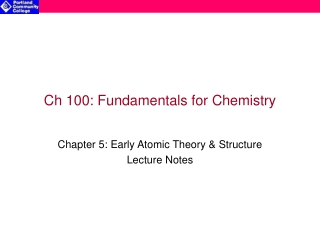Download PresentationCh 100: Fundamentals for Chemistry

Loading in 2 Seconds...

# Ch 100: Fundamentals for Chemistry

Download Presentation## Ch 100: Fundamentals for Chemistry

- - - - - - - - - - - - - - - - - - - - - - - - - - - E N D - - - - - - - - - - - - - - - - - - - - - - - - - - -
##### Presentation Transcript

1. Ch 100: Fundamentals for Chemistry Chapter 5: Early Atomic Theory & Structure Lecture Notes

2. 1) Earth 1) Cold 3) Air 3) Hot 2) Water 2) Moist 4) Fire 4)Dry Early Model of Matter: Aristotle (384-322 BC) • Introduced observation as an important step in understanding the natural world • According to his model of nature, all forms of matter are mixtures of one of 4 basic “elements”: • All matter has one or more of 4 basic “qualities”: According to Aristotle:Any substance could be transformed into any other substance by altering the relative proportion of these elements and qualities (i.e. lead to gold)

3. Dalton’s Atomic Theory • Each element consists of individual particles called atoms • Atoms can neither be created nor destroyed • All atoms of a given element are identical • Atoms combined chemically in definite whole-number ratios to form compounds • Atoms of different elements have different masses

4. The Modern Atomic Model According to our modern model of the matter, the atom has 2 primary regions of interest: • Nucleus • Contains protons & neutrons (called nucleons, collectively) • Establishes most of the atom’s mass • Mass of 1 neutron = 1.675 x10-27 kg • Mass of 1 proton = 1.673 x10-27 kg • Small, dense region at the center of the atom • The radius of the nucleus ~ 10-15 m (1 femtometer) • The Electron Cloud • Contains electrons • Mass of 1 electron = 9.109 x10-31 kg • Establishes the effective volume of the atom • The radius of the electron cloud ~ 10-10 m (1 Angstrom) • Determines the chemical properties of the atom • During chemical processes, interactions occur between the outermost electrons of each atom • The electron properties of the atom will define the type(s) of interaction that will take place

5. Structure of the Atom

6. What holds the atom together? • Electromagnetic interaction(a.k.a. electric force) holds the electrons to the nucleus • The negative charge (-) of the electrons are attracted to the positive charge (+) of the nucleus • Strong interaction(a.k.a. strong force) holds the nucleons together within the nucleus • The positive charge of the protons repel each other • All nucleons, protons and neutrons, possess a STRONG attraction to each other that overcomes the protons’ mutual repulsion

7. Electric Charge • Electric charge is a fundamental property of matter • We don’t really know what electric charge is but we do know that there are 2 kinds: • Positive charge (+) • Negative charge (-) • Opposite charge polarity is attractive: + attracts - • Same charge polarity is repulsive: + repels + and – repels – • The magnitude of electric charge (q) is the same for protons and electrons: • The charge of a proton (qproton) or electron (qelectron) is the smallest amount that occurs in nature, it is called the quantum of charge: • qproton = +1.602 x 10-19 Coulombs (or 1+) • qelectron = -1.602 x 10-19 Coulombs (or 1-)

8. Ions • Atoms (or molecules) that have gained or lost one or more electrons • Ions that have lost electrons are called cations • Ions that have gained extra electrons are called anions • Ionic compounds have both cations and anions (so that their net charge is zero)

9. - + + + - - Ions (cont.) • Ions are electrically charged atoms and thus carry electric charge: • The electric charge of an ion is due to the imbalance of electrons and protons • When an atom has lost one or more of its electrons it carries a positive charge “1+” for each electron that is lost • When an atom has gained one or more of its electrons it carries a positive charge “1-” for each excess electron that is gained • When an atom/molecule is an ion, its charge must be specified: • Sodium ion: Na+ • Chloride ion: Cl- • Hydroxide ion: OH- • Notes on Electric Charge: • Opposite charges attract • Like charges repel

10. Atomic Bookkeeping • Atomic number (Z) • The number of protons in an atom or ion • The number that defines the identity of the atom • Mass number (A) • The number of protons & neutrons in a specific atom or isotope • The number that represents the mass of an atom To determine number of neutrons in an atom: # of neutrons = (Mass #) – (Atomic #) Or # of neutrons = A - Z

11. Mass # (Atomic Symbol) Atomic # 12 14 C C 6 6 Mass # vs. Atomic Mass Isotopes are the equivalent of sibling members of an element • Unique atoms of the same element with different mass numbers (i.e. they have different numbers of neutrons) • Unique isotopes are identified by their mass number Isotope notation: Example:carbon-12 ( )&carbon-14 ( ) Atomic mass • The average total mass of an element’s various naturally occurring isotopes • The unit of Atomic Mass is the Dalton (or amu) • 1 Dalton = one twelfth mass of one 12C atom = 1.661x10-27 kg Note: There 6 protons & 6 neutrons in a 12C atom but the mass of a 12C atom is actually slightly less than the combined mass of all of the nucleons individually. Where is this lost mass?It’s released as energy when the nucleons combine (bind) to form the nucleus of the atom.

12. Examples of Isotopes